Author: Oscar Cronquist Article last updated on May 04, 2022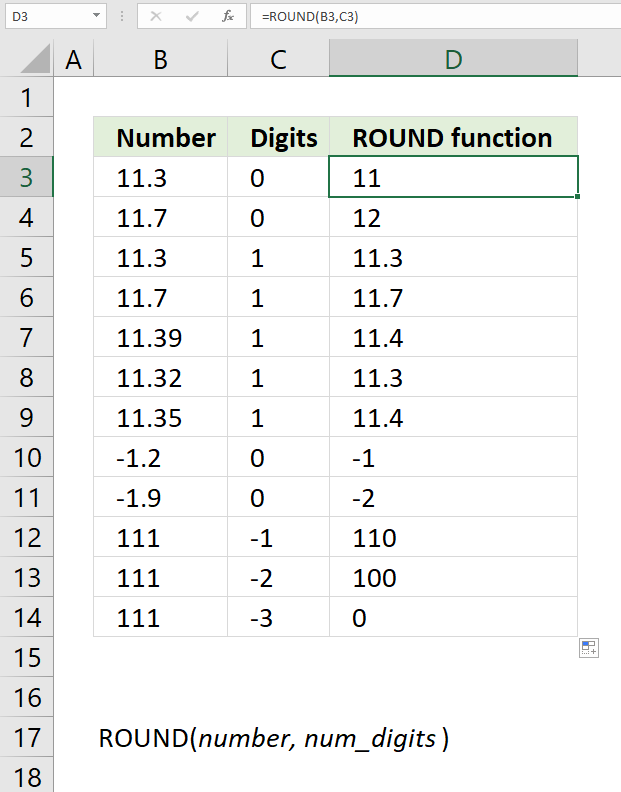The ROUND function calculates a rounded number based on the number of digits you specify.

Formula in cell D3:

=ROUND(B3, C3)

### Excel Function Syntax

ROUND(number, num_digits)

### Arguments

 number Required. The value to round. num_digits Required. A number representing the digit you want to round down to.

The ROUND function rounds

## 1. How to round a number to its nearest integer (whole number)?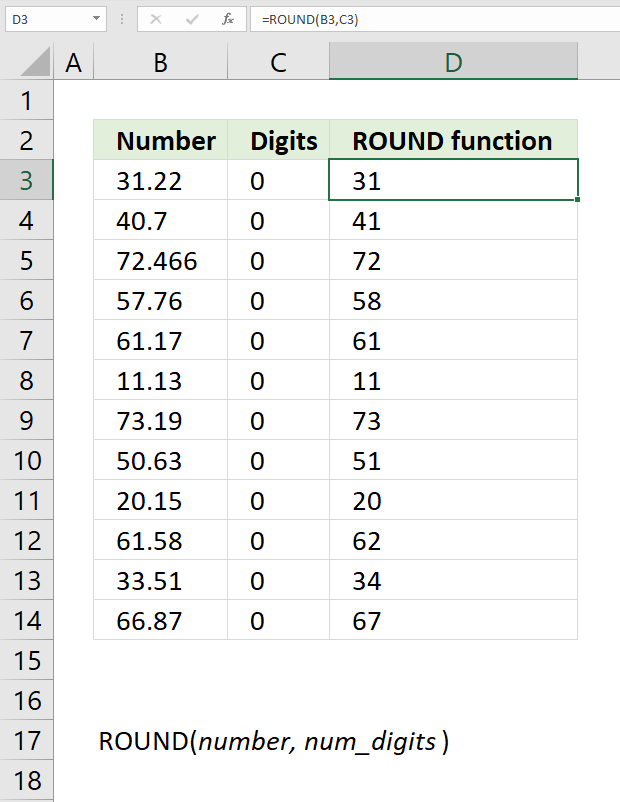Formula in cell D3:

=ROUND(B3, C3)

## 2. How to round a number to n decimals?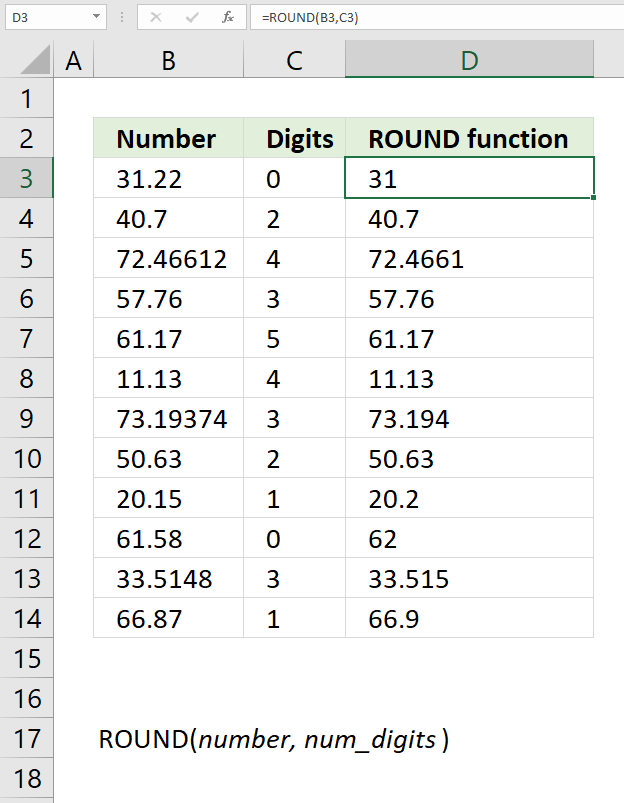Formula in cell D3:

=ROUND(B3, C3)

## 3. How to round a number to the nearest multiple of 10?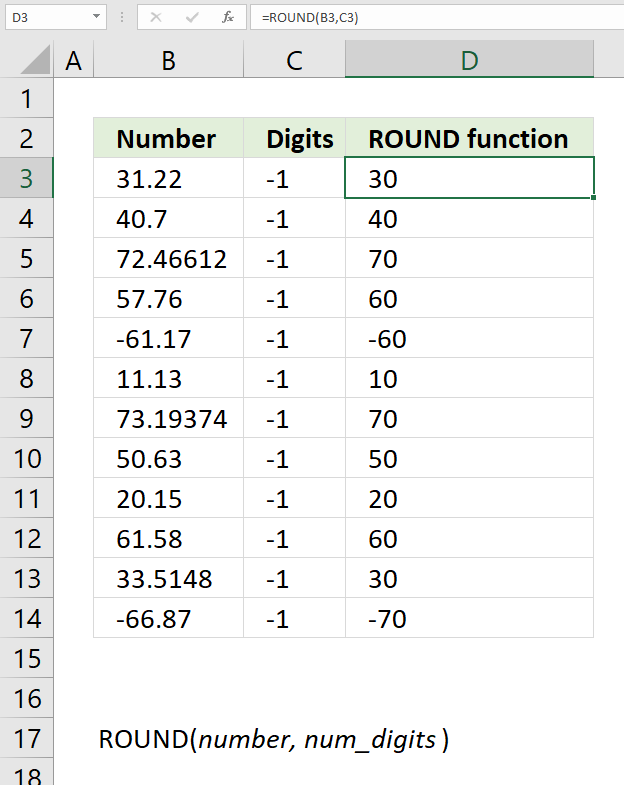Formula in cell D3:

=ROUND(B3, C3)

## 4. How to round a number to the nearest multiple of 100?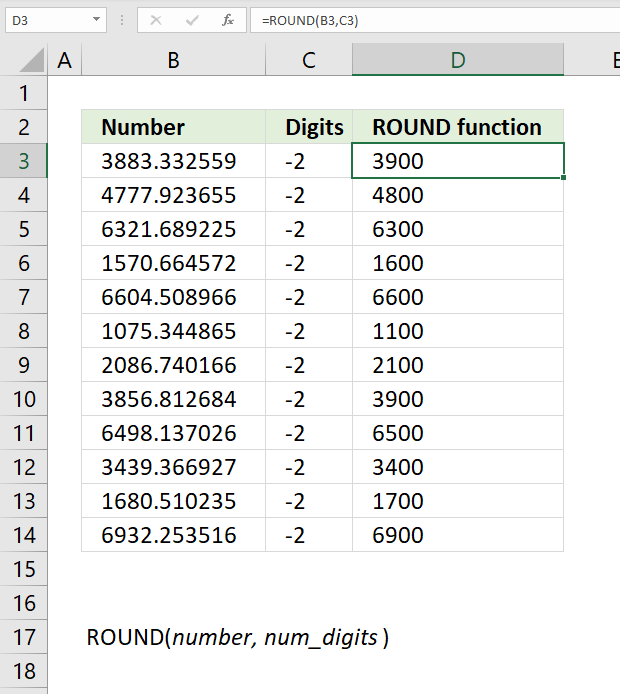Formula in cell D3:

=ROUND(B3, C3)

## 5. How to round a number to the nearest multiple of 1000?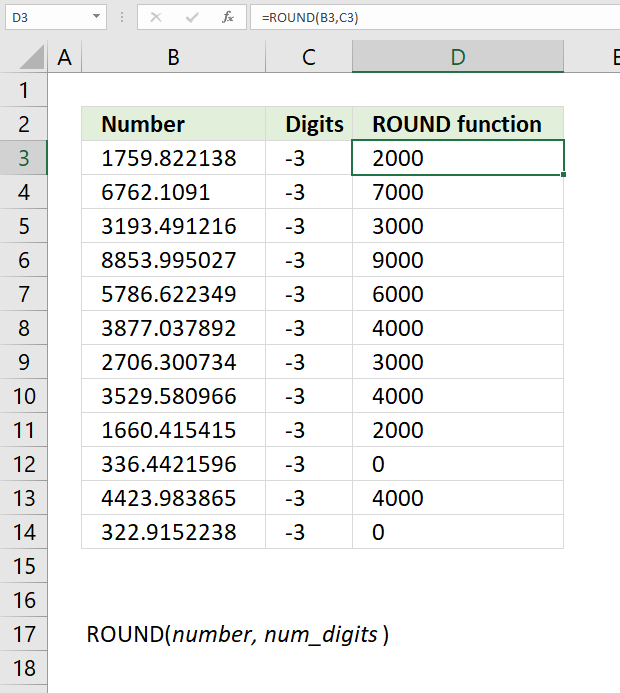Formula in cell D3:

=ROUND(B3, C3)